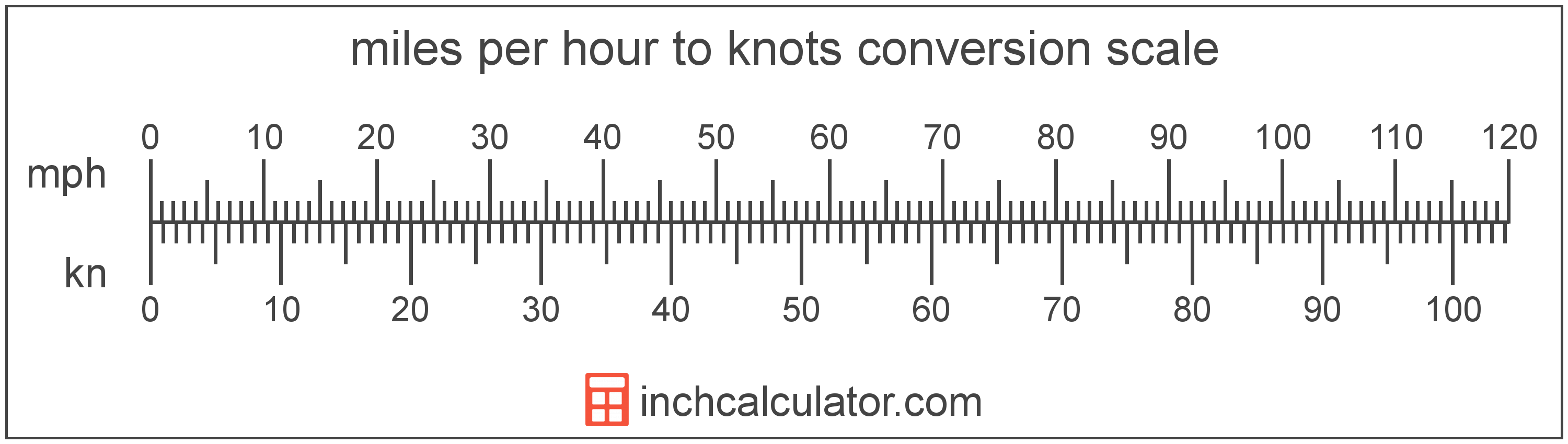# Knots to Miles per Hour Converter

Enter the speed in knots below to get the value converted to miles per hour.

Results in Miles per Hour:1 kn = 1.150779 mph

Do you want to convert miles per hour to knots?

## How to Convert Knots to Miles per Hour

To convert a measurement in knots to a measurement in miles per hour, multiply the speed by the following conversion ratio: 1.150779 miles per hour/knot.

Since one knot is equal to 1.150779 miles per hour, you can use this simple formula to convert:

miles per hour = knots × 1.150779

The speed in miles per hour is equal to the speed in knots multiplied by 1.150779.

For example, here's how to convert 5 knots to miles per hour using the formula above.
miles per hour = (5 kn × 1.150779) = 5.753897 mphKnots and miles per hour are both units used to measure speed. Calculate speed in knots or miles per hour using our speed calculator or keep reading to learn more about each unit of measure.

## What is a Knot?

One knot is equal to a speed of one nautical mile per hour, or one minute of latitude per hour.

Knots can be abbreviated as kn, and are also sometimes abbreviated as kt. For example, 1 knot can be written as 1 kn or 1 kt.

## What Are Miles per Hour?

Miles per hour are a measurement of speed expressing the distance traveled in miles in one hour.

The mile per hour is a US customary and imperial unit of speed. Miles per hour can be abbreviated as mph, and are also sometimes abbreviated as mi/h or MPH. For example, 1 mile per hour can be written as 1 mph, 1 mi/h, or 1 MPH.

Miles per hour can be expressed using the formula:
vmph = dmi / thr

The velocity in miles per hour is equal to the distance in miles divided by time in hours.

## Knot to Mile per Hour Conversion Table

Table showing various knot measurements converted to miles per hour.
Knots Miles Per Hour
1 kn 1.1508 mph
2 kn 2.3016 mph
3 kn 3.4523 mph
4 kn 4.6031 mph
5 kn 5.7539 mph
6 kn 6.9047 mph
7 kn 8.0555 mph
8 kn 9.2062 mph
9 kn 10.36 mph
10 kn 11.51 mph
11 kn 12.66 mph
12 kn 13.81 mph
13 kn 14.96 mph
14 kn 16.11 mph
15 kn 17.26 mph
16 kn 18.41 mph
17 kn 19.56 mph
18 kn 20.71 mph
19 kn 21.86 mph
20 kn 23.02 mph
21 kn 24.17 mph
22 kn 25.32 mph
23 kn 26.47 mph
24 kn 27.62 mph
25 kn 28.77 mph
26 kn 29.92 mph
27 kn 31.07 mph
28 kn 32.22 mph
29 kn 33.37 mph
30 kn 34.52 mph
31 kn 35.67 mph
32 kn 36.82 mph
33 kn 37.98 mph
34 kn 39.13 mph
35 kn 40.28 mph
36 kn 41.43 mph
37 kn 42.58 mph
38 kn 43.73 mph
39 kn 44.88 mph
40 kn 46.03 mph

## References

1. NASA, Knots Versus Miles per Hour, https://www.grc.nasa.gov/WWW/K-12/WindTunnel/Activities/knots_vs_mph.html
2. Wikipedia, Miles per hour, https://en.wikipedia.org/wiki/Miles_per_hour